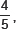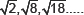Class X Math
Sample Paper for Arithmetic Progressions
1.   Write the common difference of an A.P. whose nth term is 3n + 5.
2.   Write the value of x for which x + 2, 2x, 2x + 3 are three consecutive terms of an A.P.
3.   For what value of k, are the numbers x, (2x + k) and (3x + 6) three consecutive terms of an A.P.?
4.   Ifa, 2 are three consecutive terms of an A.P., then find the value of a?
5.   For what value of p are 2p – 1, 7 and 3p three consecutive terms of an A.P.?
6.   For what value of p are 2p + 1, 13 and 5p – 3 three consecutive terms of an A.P.?
7.   Find the next term of the A.P.8.   Which term of the A.P.:
21, 18, 15, .... is zero?
9.   If the sum of first 7 terms of an A.P. is 49 and that of first 17 terms is 289, find the sum of n terms.
10.   Find the sum of all three digit numbers which are divisible by 7.
11.   Find the sum of all the three digit numbers which are divisible by 9.
12.   If Sn the sum of n terms of an A.P. is given by Sn = 3n2 – 4n, find the nth term.
13.   The sum of 4th and 8th terms of an A.P. is 24, and the sum of 6th and 10th terms is 44. Find the A.P.
14.   The Sum of n terms of an A.P. is 5n2 – 3n. Find the A.P. Hence find its 10th term.
15.   The sum of 4th and 8th terms of an A.P. is 24 and the sum of 6th and 10th terms is 44. Find the first three terms of the A.P.